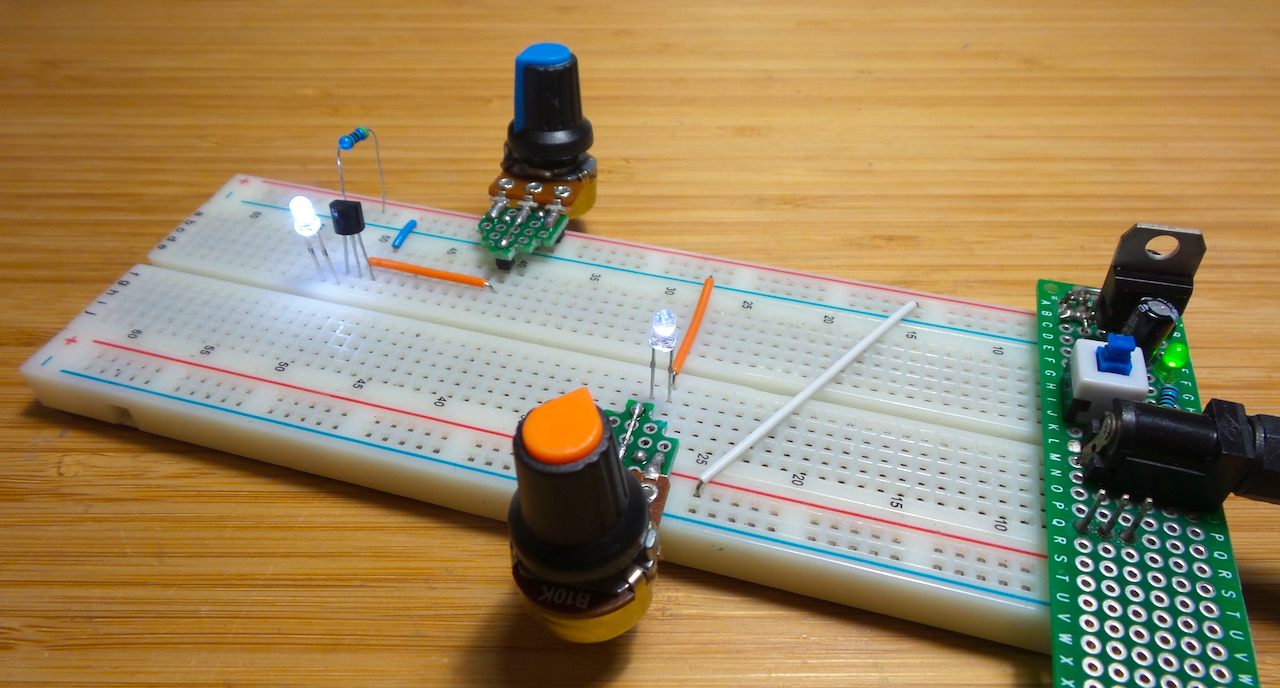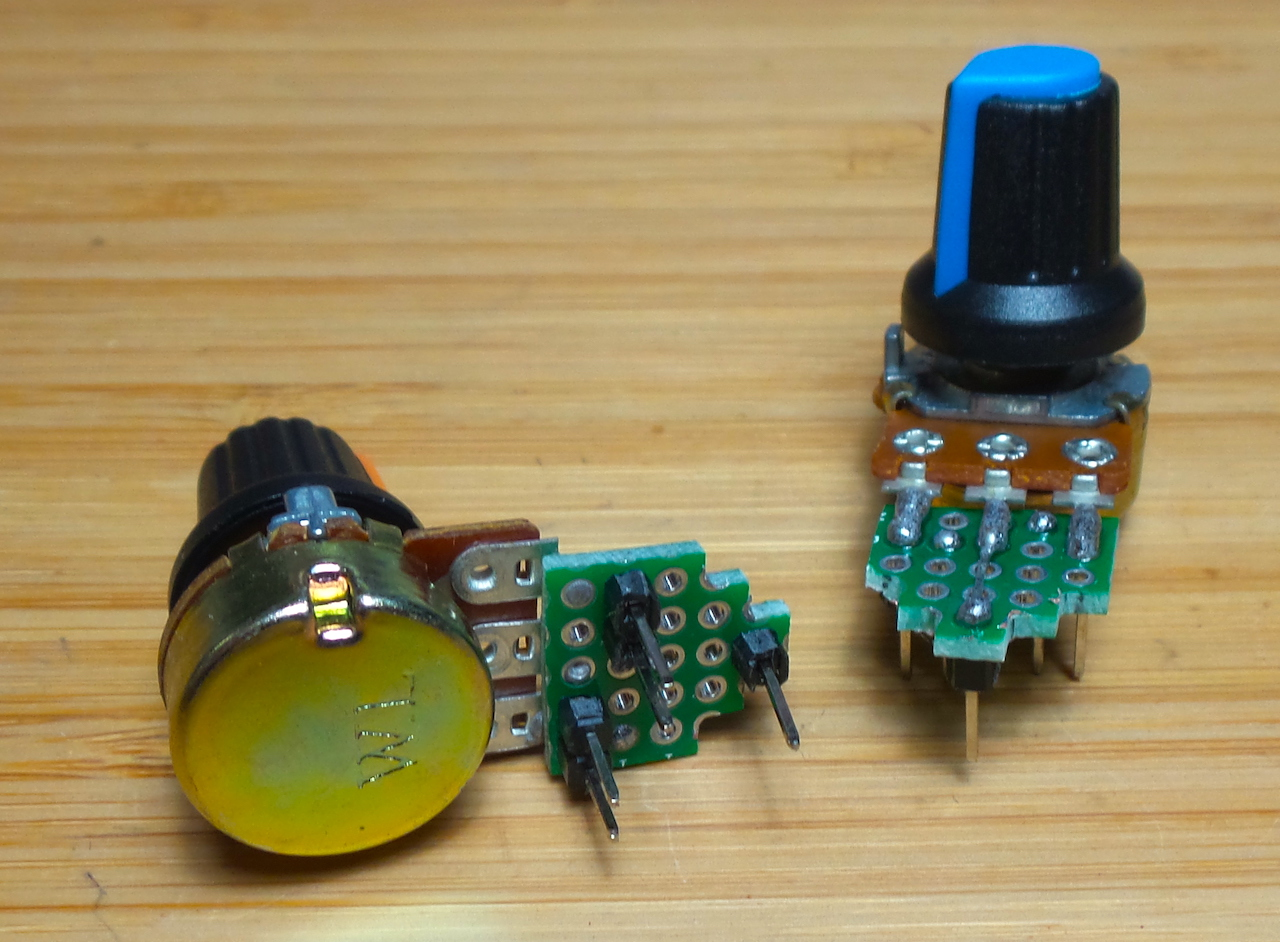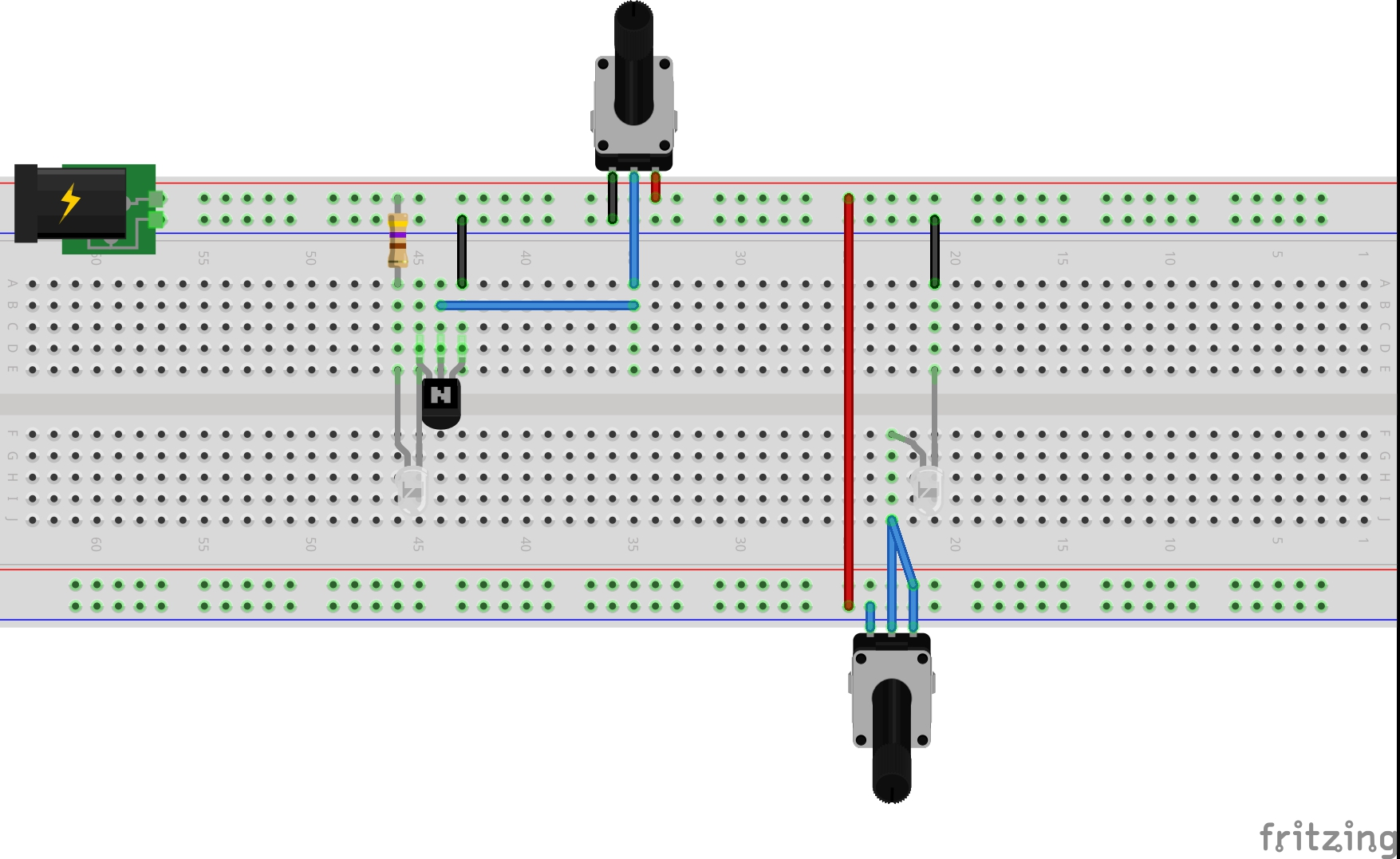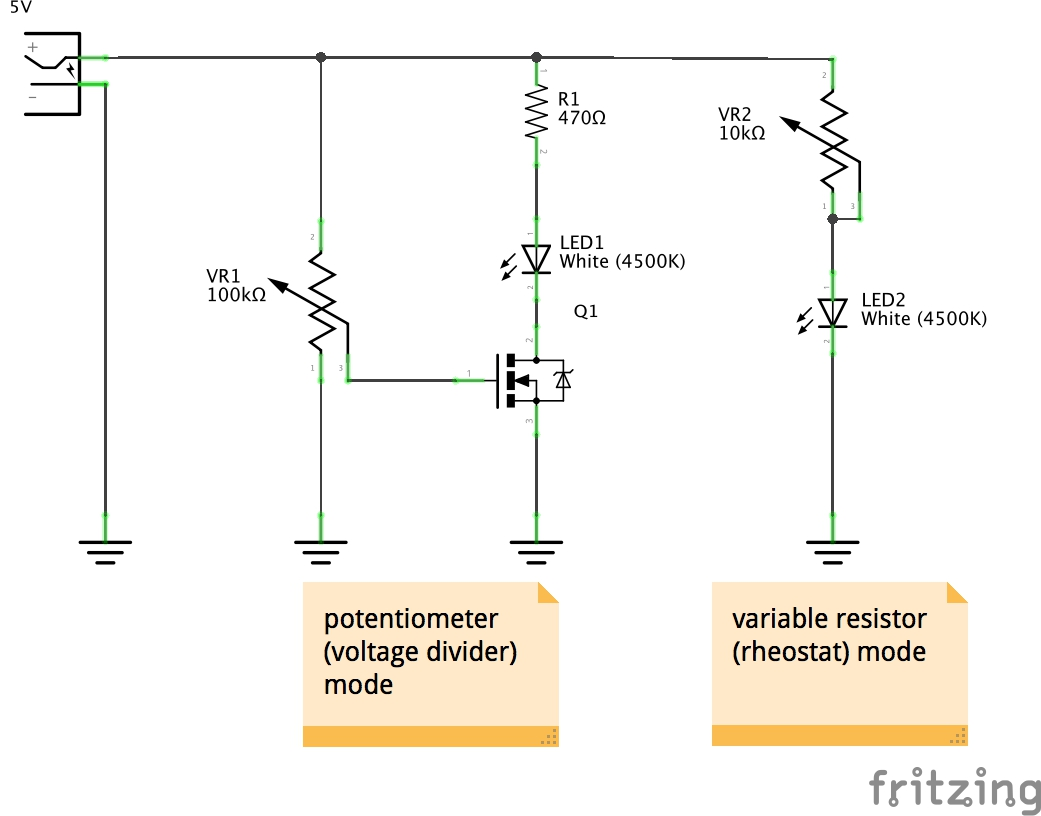### Project Notes

Making some breadboard-compatible potentiometers and covering some basics of terminology, Ohm’s Law and voltage dividers.A simple little project to make breadboard prototyping just a little more efficient..

I’ll often want to throw a potentiometer on a breadboard, usually to provide an adjustable voltage reference somewhere between ground and the positive power rail. But it can be a bit of a pain:

• standard components with the 5.5mm shaft can be forced into a breadboard, but it is a tight fit and is probably dmaging the breadboard recepticles
• trim pots can plug into a breadboard, but the connection is often insecure (they are liable to pop themselves out)

So I finally had enough of this and decided to mount some pots on some protoboard with pins that plug snuggly into a breadboard.

### Terminology

• potentiometer: the familiar three-terminal component with an adjustable contact (the wiper) that forms a voltage divider. Variants:
• Linear potentiometers: with a slider
• Trimpots/trimmer pots:
• variable resistor aka rheostat: a two terminal component with adjustable resistance between the terminals

In most applications these days, the role of variable resistor or rheostat is usually filled by using only two terminals of a potentiometer

### Construction

I built two modules (10kΩ, 100kΩ linear type B).

They are mounted on a 5 hole * 5 hole piece of protoboard. Pins on the board are configured to connect by default:

• the end terminals of the potentiometer are seated on the power rails of a bradboard
• wiper plugs into a row on the breadboard

Some knobs attached for extra bling.### Demonstration Circuit

The circuit demonstrates the modules being used in both roles:

• as a rheostat to vary the resistance and therefore current supplied to an LED
• as a potentiometer voltage divider to control the gate voltage of an n-channel MOSFET to provide low-side switching of an LED### Experiements in Basic Electronics

Potentiometers are one of the first components that students of basic electronics may encounter, and they are particularly useful for exploring and understanding fundamental concepts such as Ohm’s Law and voltage dividers.

#### Ohm’s Law

Ohm’s Law:

``````V = IR
``````

Where:

• V = voltage (volts, V)
• I = current (amps, A)
• R = resistance (ohms, Ω)

A simple experiment to demonstrate the reliability of Ohm’s Law:

• connect a voltage across a potentiometer in rheostat mode set to roughly mid-point
• measure with a multimeter:
• voltage across the rheostat
• current through the rheostat
• resistance of the rheostat at this particular setting (take it out of circuit to make this measurement)
• substituting any two of these measurements in the equation `V = IR` should yield the third term close to the measured value
• repeat with the rheostat adjusted to other positions
• but be careful of short circuit condition - for this reason, a good idea to have another resistor in series with the rheostat

#### The Voltage Divider

Two resistors in series (R1, R2) form a voltage divider. The voltage at the join between R1 and R2 is given by the equation:

``````V = R2 / (R1 + R2)
``````

This equation is actually derived form Ohm’s Law.

A potentiometer is in effect a voltage divider in a single package, with the wiper tapping the connection of the upper and lower resistaances (R1, R2 respectively).

A simple experiment to demonstrate the reliability of the Voltage Divider equation:

• connect a voltage across a potentiometer. measure this voltage
• with the wiper at various positions, measure with a multimeter:
• the voltage at the wiper
• the resistance between wiper and terminal connected to the positive voltage (R1)
• the resistance between wiper and terminal connected to the negative voltage (R2)
• take the potentiometer out of circuit to make the resistance measurements
• substituting any two of these measurements in the equation `V = R2 / (R1 + R2)` should yield the third term close to the measured value